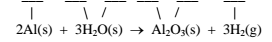# Problem: Consider the following reactions: 1.   2Al(s) + 3/2 O2 (g) → Al2O3 (s)        ΔH° = -1669.8 kJ/mol   2.   H2 (g) + 1/2 O2 (g) →  H2O (l)         ΔH° =    -285.8 kJ/mol 3.   H2O (s) → H2O (l)                           ΔH° =       6.01 kJ/mol For which, if any, reactions does ΔH° correspond to a molar enthalpy of formation?    Write the oxidation numbers in the spaces provided.

###### FREE Expert Solution
91% (191 ratings)
###### Problem Details

Consider the following reactions:

1.   2Al(s) + 3/2 O2 (g) → Al2O3 (s)        ΔH° = -1669.8 kJ/mol

2.   H2 (g) + 1/2 O2 (g) →  H2O (l)         ΔH° =    -285.8 kJ/mol

3.   H2O (s) → H2O (l)                           ΔH° =       6.01 kJ/mol

For which, if any, reactions does ΔH° correspond to a molar enthalpy of formation?

Write the oxidation numbers in the spaces provided.What scientific concept do you need to know in order to solve this problem?

Our tutors have indicated that to solve this problem you will need to apply the Enthalpy of Formation concept. You can view video lessons to learn Enthalpy of Formation. Or if you need more Enthalpy of Formation practice, you can also practice Enthalpy of Formation practice problems.

What is the difficulty of this problem?

Our tutors rated the difficulty ofConsider the following reactions: 1.   2Al(s) + 3/2 O2 (g) ...as medium difficulty.

How long does this problem take to solve?

Our expert Chemistry tutor, Jules took 2 minutes and 29 seconds to solve this problem. You can follow their steps in the video explanation above.

What professor is this problem relevant for?

Based on our data, we think this problem is relevant for Professor Dixon's class at UCF.